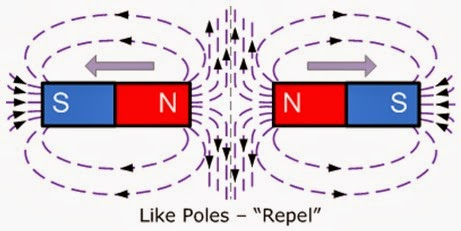# Electromagnetism (Physics)Hans Christian Orated was the father of electromagnetism. His famous experiments refueled the connection between electricity and magnetism. The number of magnetic lines passing through the Area ‘A’ is known as flux (φ). If B is magnetic flux density then BAφ=or φ= BA.When the reference plane makes an angle with the field, then φ= BA cosθ If q use the change moving with velocity v in magnetic field of indentation B then the force acting on the change is F = q νB sinθ.

The direction of force can be measured by the light hand rule. Electric motor is a device which can be converted electric energy into mechanical energy. Faraday’s experiments tell about the link between change of magnetic flux and a current generation in the coil.